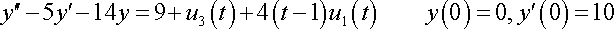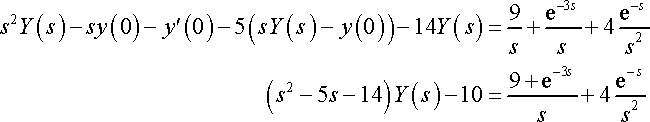# Laplace transforms, heaviside step functions

Hey guys! I just have a question regarding some notes I've been looking at and trying to understand...

## Homework StatementTaking the transform:Just wondering, can someone explain to me why the e-3s/s is positive, and not negative because it is originally -u3(t)...

Another quick one:

Write this in terms of Heaviside function:

f(t) =
{ 0, 0 <= t <= pi
{ -sin3t, t >= pi

1) So you would get f(t) = -(upit)sin3t

And somehow it ends up as f(t) = (upit)sin(3(t-pi))
Can someone explain this step as well? Just what happened to the negative in front of it, and why it became 3(t-pi)

Thanks!

vela
Staff Emeritus
Homework Helper
Hey guys! I just have a question regarding some notes I've been looking at and trying to understand...

## Homework StatementTaking the transform:Just wondering, can someone explain to me why the e-3s/s is positive, and not negative because it is originally -u3(t)...
It's probably just a typo in the original problem.

Another quick one:

Write this in terms of Heaviside function:

f(t) =
{ 0, 0 <= t <= pi
{ -sin3t, t >= pi

1) So you would get f(t) = -(upit)sin3t

And somehow it ends up as f(t) = (upit)sin(3(t-pi))
Can someone explain this step as well? Just what happened to the negative in front of it, and why it became 3(t-pi)
Try expanding sin[3(t-pi)] using the angle addition formula for sine. The reason it was written this way is probably because it's now in the form where the time-shift property of the Laplace transform can be applied.

#### Attachments

Thanks for the first one that makes sense.

For the second one, I attempted using the identity and get:

f(t) = -upi(t)sin(3t)
= -L{upi(t)sin3(t-pi+pi)}
= -L{upi(t)[sin3(t-pi)cos(3pi) + sin(3pi)cos3(t-pi)]}
taking the transform:
= -e-pi*s(3cos(3pi) + 3s*sin(3pi) / s2 + 9

Hmm I don't understand how it is suppose to be just upi(t)sin(3(t-pi)) = e-pi*s(3) / (s2 + 9)(s2 + 1)

What happened when you add the pi and subtract it, and what happened to the negative in front of it all?

vela
Staff Emeritus